Next: 2.2.1 K-means Up: 2. Modeling of data Previous: 2.1.2 Probabilistic PCA

# 2.2 Vector quantization

Vector quantization describes a pattern set using a reduced number of so-called code-book' vectors. We assume again that n training patterns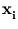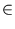IRd are given. Let m < n be the number of code-book vectors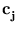IRd. In the final state, each training pattern is assigned to one code-book vector. The optimal position of code-book vectors is usually gained by finding the minimum of the sum E of squared distances2.2 between each code-book vector and its assigned patterns,

 E =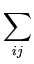P(j|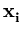)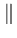-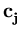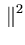. (2.12)

P(j|) is the probability thatbelongs to. For the final state, P(j|) = 1 ifis assigned to, and P(j|) = 0 otherwise. A pattern is assigned to the code-book vector that has the smallest Euclidean distance to that pattern. Thus, the code-book vectors induce a Voronoi tessellation of space (figure 2.2). In each of the separated regions, the position of the code-book vector is the center-of-mass of the local pattern distribution (otherwise (2.12) cannot be minimal).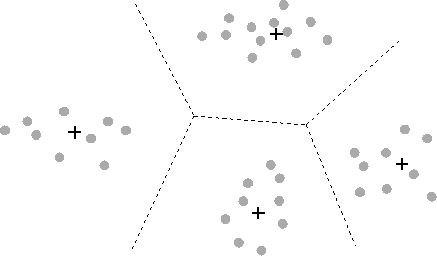The difficulty in finding the optimal {} is that E has many local minima. No general solution exists. Instead, various iterative algorithms exist that find approximate solutions. The algorithms can be divided into those that use hard-clustering and those that use soft-clustering. In hard-clustering, P(j|) is binary (throughout the iteration), and eachcan be only assigned to one code-book vector. In soft-clustering, P(j|) can take any value in the interval [0;1].

The algorithms can be further divided into on-line and batch versions. On-line versions update code-book vectors in each iteration step based on just one (randomly drawn) training pattern(as for the self-organizing map, section 1.5.5). The update rule is usually written as(t + 1) =(t) +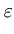(t)P(j|)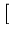-(t)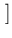. (2.13)

The change ofis in the direction of the negative gradient of (2.12).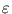(t) is a learning rate, which can depend on time. In contrast, batch versions use all training patterns for each step. Here, the algorithm alternates between computing all P(j|) based on a given distribution {}, and optimizing allgiven P(j|). This algorithm is a variant of the expectation maximization algorithm (Dempster et al., 1977). The maximization' step is a minimization of the error E (which maximizes the likelihood, see section 2.3.1).

The following sections describe the hard-clustering algorithm k-means' and provide more details about soft-clustering algorithms. Two examples are described: deterministic annealing' and `Neural Gas'.

SubsectionsNext: 2.2.1 K-means Up: 2. Modeling of data Previous: 2.1.2 Probabilistic PCA
Heiko Hoffmann
2005-03-22